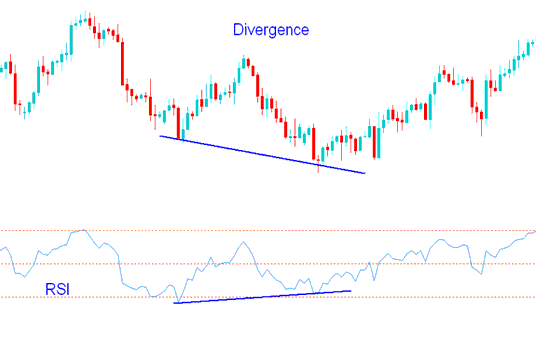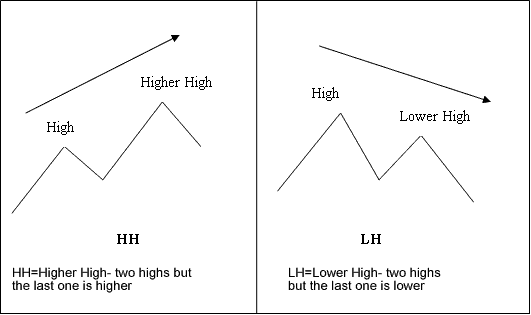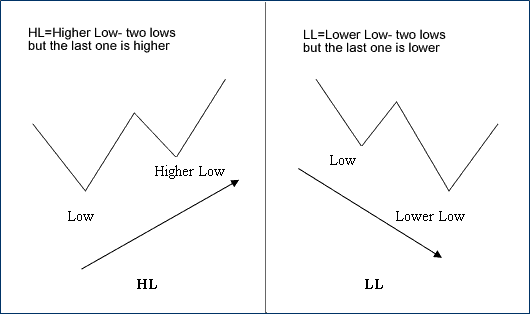# RSI Indicator Divergence Trading Setups - Bullish Divergence RSI and Bearish Divergence RSI

Forex Divergence is one of the trade setups used by traders. It involves looking at a forex chart and one more forex technical indicator. For our example we shall use the RSI indicator.

To spot this forex divergence trading setup find two chart points at which price makes a new swing high or a new swing low but the RSI indicator does not, indicating a divergence between price and momentum.

RSI Forex Divergence Example:

In the forex chart below we identify two chart points, point A and point B (swing highs)

Then using RSI indicator we check the highs made by the forex RSI indicator, these are the highs that are directly below the forex Chart points A and B.

We then draw one line on the forex chart and another line on the RSI indicator.RSI Divergence Forex Trading Setup - Forex Divergence Trading using RSI Indicator - Bullish Divergence RSI and Bearish Divergence RSI

How to spot forex divergence

In order to spot this forex divergence trading setup we look for the following:

HH = Higher High - two highs but the last one is higher

LH = Lower High - two highs but the last one is lower

HL = Higher Low - two lows but the last one is higher

LL = Lower Low - two lows but the last one is lower

First let us look at the illustrations of these forex trading termsDivergence Forex Trading Terms Definition - Bullish Divergence RSI and Bearish Divergence RSIForex Divergence Trading Terms Definition Examples - Bullish Divergence RSI and Bearish Divergence RSI

There are two types of forex divergence trading setups:

1. Classic Divergence
2. Hidden Divergence

Forex Contest Limited Time

UpTo 5K Bonus Limited Time